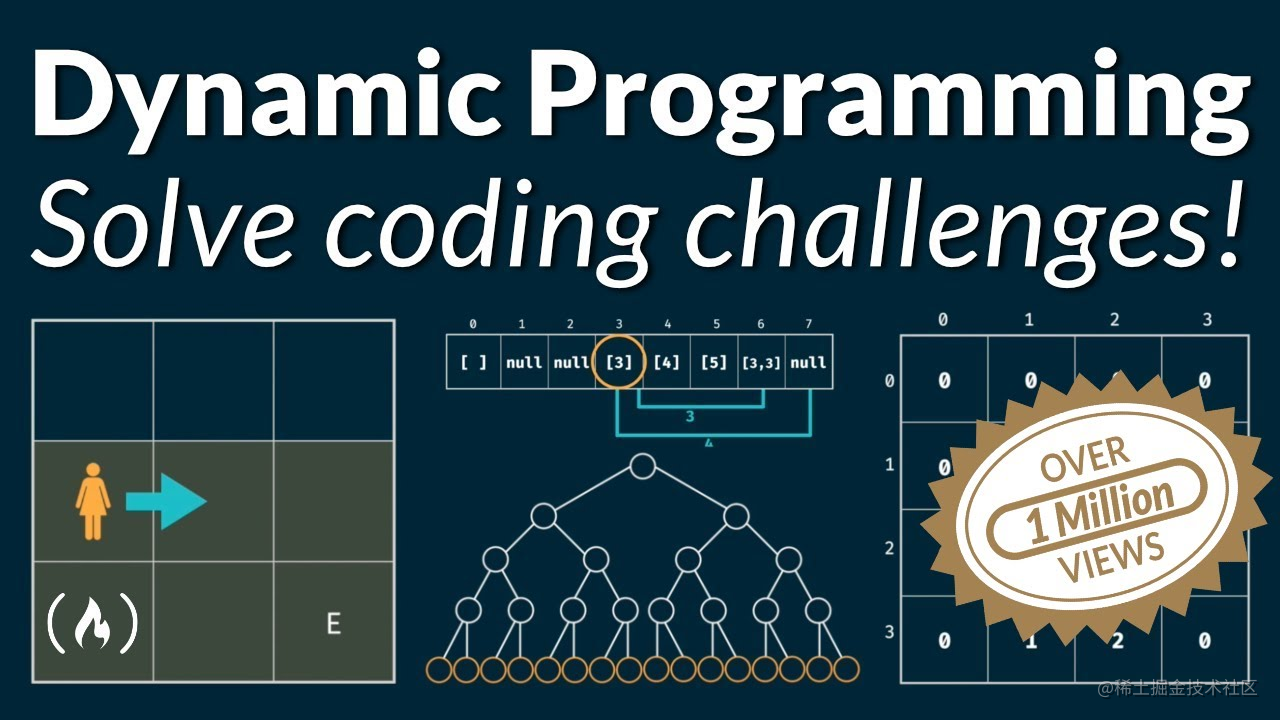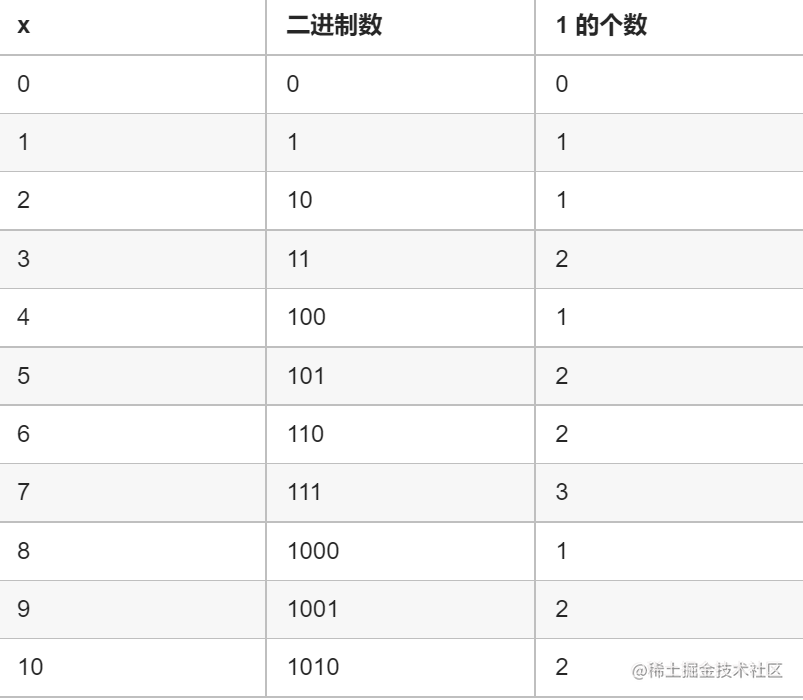# 算法简单题，吾辈重拳出击 - 前 n 个数字二进制中 1 的个数OK，简单温故思路，再开始本篇题目：前 n 个数字二进制中 1 的个数

``````输入: n = 2

0 --> 0
1 --> 1
2 --> 10

``````输入: n = 5

0 --> 0
1 --> 1
2 --> 10
3 --> 11
4 --> 100
5 --> 101

## 第一反应

n=2，就要写出 0、1、2 的二进制，分别是 0 、1、10，分别有1的个数：0、1、1

n=3，就要写出 0、1、2、3 的二进制，分别是0、1、10、11，分别有1的个数：0、1、1、2

n=5 => [0,1,1,2,1,2]

......

## 第二反应

x = x & (x-1) 用于去除整数 x 二进制最右边的 1，递进移除，进行计数。

``````let x = 5;

x = 5 & 4;
// 5:     00000000000000000000000000000101
// 4:     00000000000000000000000000000100

// 00000000000000000000000000000100

x = x & 3
// x:       00000000000000000000000000000100
// 3:       00000000000000000000000000000011

// 00000000000000000000000000000000

``````var countOnes = function(x){
let count = 0;
while(x > 0){
x &= x - 1;
// 统计次数
count++;
}
return count;
}

## 第三反应

``````var countBits = function(n) {
let res = []
let countOnes = function(x){
let count = 0;
while(x > 0){
x &= x - 1;
// 统计次数
count++;
}
return count;
}
for(let i = 0;i<=n;i++){
res.push(countOnes(i))
}

return res
};## 第四反应

DP 就是将大问题抽象为一个小的具体问题，用公式表达。 通常由后向前导，遍历一次，时间复杂度更小。

``````// 比如正整数 5 ，小于等于 5 的最大正整数，且它是 2  的整数次幂，且只有最高位是 1，其余都为 0 的是数字 4；

bits = bits [5 - 4] + 1 = bits + 1 = 2

// 比如正整数 8，小于等于  7 的最大正整数，且它是 2  的整数次幂，且只有最高位是 1，其余都为 0 的是数字 4

bits = bits [7 - 4] + 1 = bits + 1 = 3

// 比如正整数 8，小于等于  8 的最大正整数，且它是 2  的整数次幂，且只有最高位是 1，其余都为 0 的是数字 8

bits = bits [8 - 8] + 1 = bits + 1 = 1y 的二进制表示中只有最高位是 1，其余都是 0，因此 y & (y−1)=0

for 循环一层 x，当 x & (x−1)=0 的时候，更新 y 值，循环完毕，y 值肯定是最大的那个。

``````var countBits = function(n) {
let bits = new Array(n + 1).fill(0) // 结果数组要多一位
let y = 0
for(let i = 1; i <= n; i++) {
if ((i & (i - 1)) == 0) {
y = i;
}
bits[i] = bits[i - y] + 1;
}
return bits
}

## 第五反应

i & (i-1) 能将整数i的二进制形式最右边的1变为0

``````var countBits = function (n) {
let res = new Array(n + 1).fill(0);
for (let i = 1; i <= n; i++) {
res[i] = res[i & (i - 1)] + 1;
}
return res;
};

## 第六反应

OK，以上便是本篇分享。点赞关注评论，为好文助力👍